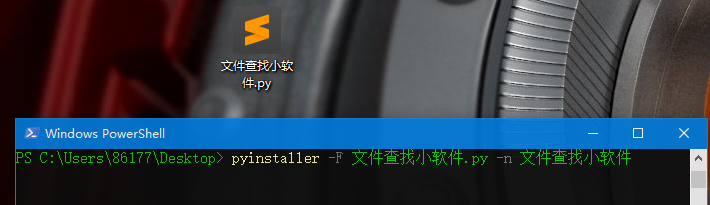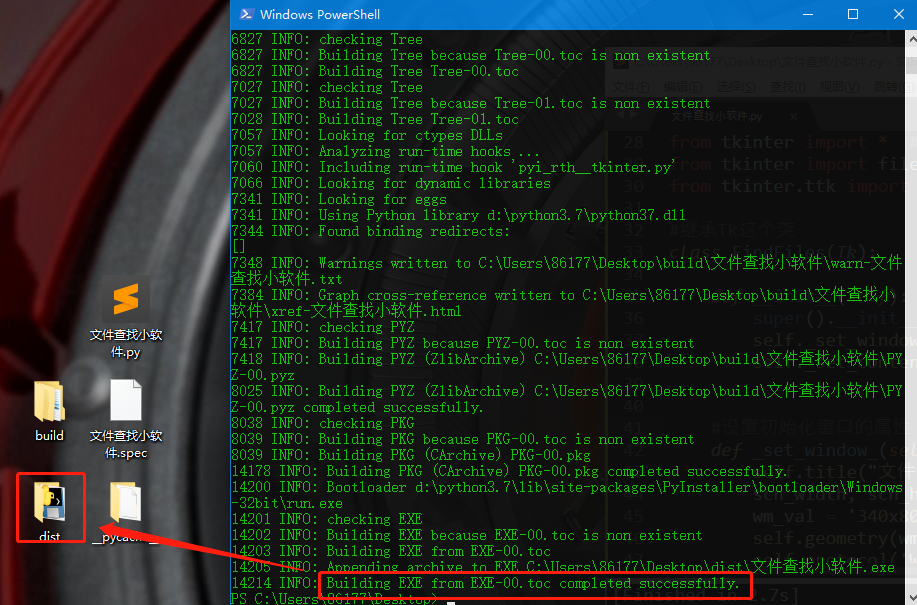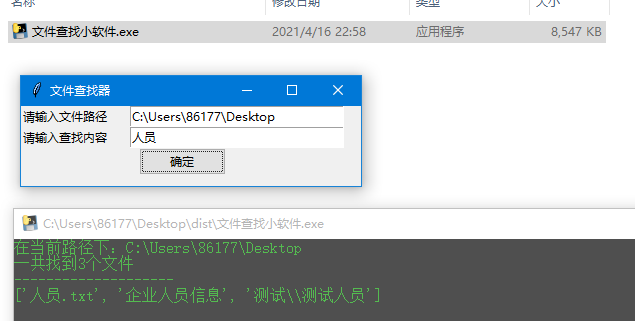### 电脑指定路径下文件查找，并封装为可执行程序

`````` 知识梳理不易，请尊重劳动成果，文章仅发布在CSDN网站上	，在其他网站看到该博文均属于未经作者授权的恶意爬取信息
``````

# 1 需求# 2 简单的模板

``````import glob
import os

def find_files(path,content='demo'):
os.chdir(path)
files = glob.glob(f'**/*{content}*', recursive = True)
print(f'在当前路径下：{path}\n一共找到{len(files)}个文件')
print('-'*20)
print(files)

find_files(r'C:\Users\86177\Desktop','人员')
``````

• 第一个是文件数据，要查找的文字是在开头的位置、
• 第二个数据是空文件夹 ，要查找的文字内容在中间、
• 第三个就是一个文件夹中的文件，除去后缀名，要查找的内容是在末尾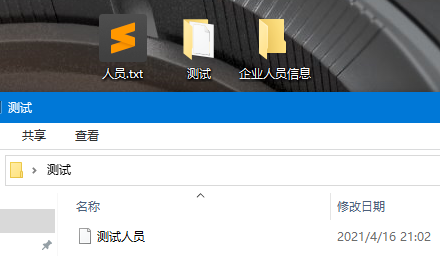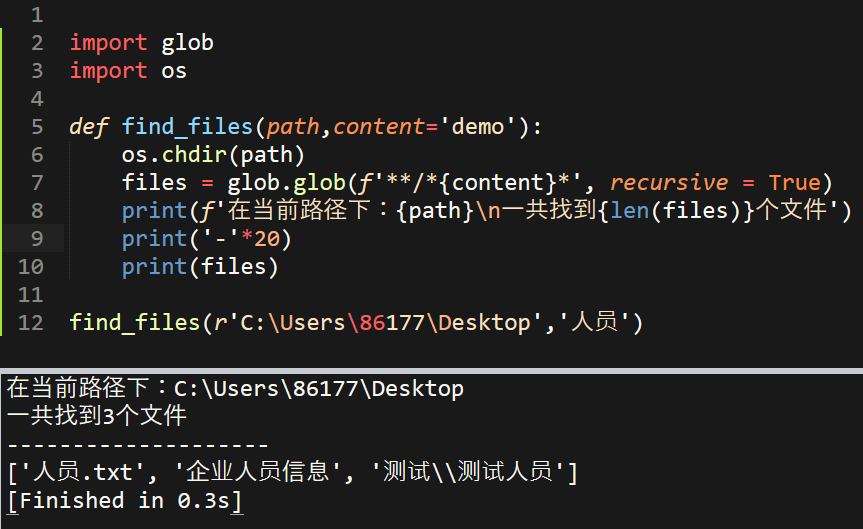# 3 代码优化并设计可视化界面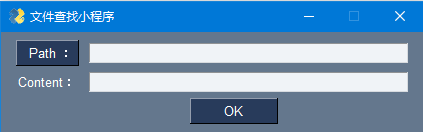``````import PySimpleGUI as sg

def find_files(path,content='demo'):
os.chdir(path)
files = glob.glob(f'**/*{content}*', recursive = True)
print(f'在当前路径下：{path}\n一共找到{len(files)}个文件')
print('-'*20)
print(files)

layout = [
[sg.FolderBrowse('  Path ：'),sg.InputText()], #第一行添加一个路径选择按钮和路径输入框，可以通过按钮选择也可手动输入
[sg.Text('Content：'),sg.InputText()], #第二行就是设置查找内容输入框
[sg.Text(' '*40),sg.OK(size = (10,1))] #第三问就是放置确认按钮，为了保证按钮在中间的位置	，两端可以塞空字符串
]

window = sg.Window('文件查找小程序', layout)
while True:
if event == sg.WIN_CLOSED or event == 'Exit':
break
# print(event,values) 通过输出的values，可以找到我们要的内容
find_files(values,values) #这里调用函数进行查找文件
print() #换行，方便下次内容的查找
window.close()
``````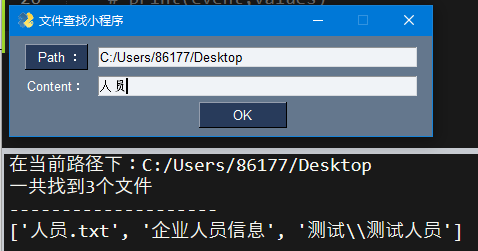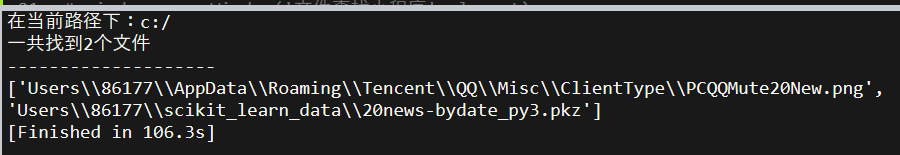# 4 再接再厉尝试tkinter及全部代码

``````from tkinter import *  #导入tkinter中所有的方法
from tkinter import filedialog, messagebox #这两个需要单独导入
from tkinter.ttk import Scrollbar, Checkbutton, Label, Button #导入ttk模块中的指定几个组件

#继承Tk这个类
class FindFiles(Tk):

def __init__(self):     #初始化自己的创建的EditorPlus类
super().__init__()  #在继承Tk这个类的基础上在自己的类中添加内容
self._set_window_() #设置程序运行主窗口
self._set_content_()

#设置初始化窗口的属性
def _set_window_(self):
self.title("文件查找器")              			     #窗口名称
scn_width, scn_height = self.maxsize() 				 #获得程序运行机器的分辨率（屏幕的长和宽）
wm_val = '340x80+{}+{}'.format((scn_width - 750) // 2, (scn_height - 450) // 2)
self.geometry(wm_val) 				   				 #将窗口设置在屏幕的中间
self.protocol('WM_DELETE_WINDOW', self.exit_editor)  #设置窗口关闭提醒

def exit_editor(self):
self.destroy() 									 #满足条件的话主窗口退出

def _set_content_(self):
Label(self, text='请输入文件路径',width=15).grid(row=0, column=0) #设置提醒输入路径的标签
self.path = Entry(self,width = 30) #设置路径输入框
self.path.grid(row=0, column=1) #放置位置
Label(self, text='请输入查找内容',width=15).grid(row=1, column=0) #设置邮箱输入标签
self.content = Entry(self,width = 30) #设置邮箱地址输入框
self.content.grid(row=1, column=1) ##放置位置
Button(self, text="确定", command=self.onclick).grid(row = 2, column = 0, columnspan = 2) #放置确定按钮

def onclick(self):
path = self.path.get()  #获取路径
content = self.content.get()  #获取邮箱地址
self.find_files(path,content)

def find_files(self,path,content='demo'):
os.chdir(path)
files = glob.glob(f'**/*{content}*', recursive = True)
print(f'在当前路径下：{path}\n一共找到{len(files)}个文件')
print('-'*20)
print(files)

if __name__ == '__main__':  #代码分块
app = FindFiles()      #类的实例化
app.mainloop()			#程序运行
``````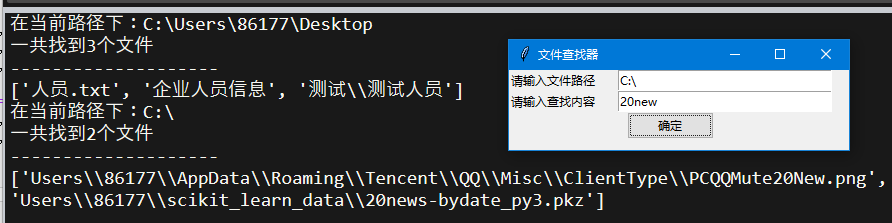``````# -*- coding:utf-8 -*-
import glob
import os
import PySimpleGUI as sg

# layout = [
# 	[sg.FolderBrowse('  Path ：'),sg.InputText()],
# 	[sg.Text('Content：'),sg.InputText()],
# 	[sg.Text(' '*40),sg.OK(size = (10,1))]
# ]

# window = sg.Window('文件查找小程序', layout)
# while True:
# 	if event == sg.WIN_CLOSED or event == 'Exit':
# 		break
# 	# print(event,values)
# 	find_files(values,values)
# 	print()
# window.close()

from tkinter import *  #导入tkinter中所有的方法
from tkinter import filedialog, messagebox #这两个需要单独导入
from tkinter.ttk import Scrollbar, Checkbutton, Label, Button #导入ttk模块中的指定几个组件

#继承Tk这个类
class FindFiles(Tk):

def __init__(self):     #初始化自己的创建的EditorPlus类
super().__init__()  #在继承Tk这个类的基础上在自己的类中添加内容
self._set_window_() #设置程序运行主窗口
self._set_content_()

#设置初始化窗口的属性
def _set_window_(self):
self.title("文件查找器")              			     #窗口名称
scn_width, scn_height = self.maxsize() 				 #获得程序运行机器的分辨率（屏幕的长和宽）
wm_val = '340x80+{}+{}'.format((scn_width - 750) // 2, (scn_height - 450) // 2)
self.geometry(wm_val) 				   				 #将窗口设置在屏幕的中间
self.protocol('WM_DELETE_WINDOW', self.exit_editor)  #设置窗口关闭提醒

def exit_editor(self):
self.destroy() 									 #满足条件的话主窗口退出

def _set_content_(self):
Label(self, text='请输入文件路径',width=15).grid(row=0, column=0) #设置提醒输入路径的标签
self.path = Entry(self,width = 30) #设置路径输入框
self.path.grid(row=0, column=1) #放置位置
Label(self, text='请输入查找内容',width=15).grid(row=1, column=0) #设置邮箱输入标签
self.content = Entry(self,width = 30) #设置邮箱地址输入框
self.content.grid(row=1, column=1) ##放置位置
Button(self, text="确定", command=self.onclick).grid(row = 2, column = 0, columnspan = 2) #放置确定按钮

def onclick(self):
path = self.path.get()  #获取路径
content = self.content.get()  #获取邮箱地址
self.find_files(path,content)

def find_files(self,path,content='demo'):
os.chdir(path)
files = glob.glob(f'**/*{content}*', recursive = True)
print(f'在当前路径下：{path}\n一共找到{len(files)}个文件')
print('-'*20)
print(files)
print()

if __name__ == '__main__':  #代码分块
app = FindFiles()      #类的实例化
app.mainloop()			#程序运行
``````

# 5 封装小程序

``````pyinstaller -F 文件查找小软件.py -n 文件查找小软件
``````